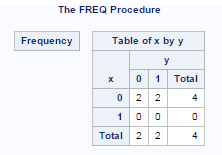## create cross table containing the row which are all zero

according to the following code

Data t;

INPUT  x        y;

cards;

0       1

0       1

0       0

0       0

;

Proc freq data=t ;

Table x*y/nopercent norow;

Run;

then i will get a results

Table of x by y

x y

0 1

0  2 2

However, if i want to get the result containing the zero number of x of 1 although it is not appear in my data set like this

Table of x by y

x y

0 1

0  2 2

1  0 0

how can i do?

i try the order statement for proc freq, but i still cannot get the result i want.

1 ACCEPTED SOLUTION

Accepted SolutionsKsharp
Super User

## Re: create cross table containing the row which are all zero

proc freq can't do that. Try proc tabulate.

### Code: Program

`Data t;INPUT  x   y;cards;0 10 10 00 0;run;data classdata; do x=0,1;  do y=0,1;   output;  end; end;run;proc tabulate data=t classdata=classdata ; class x y; table x,y /misstext='0' ; keylabel n=' ';run;   `
3 REPLIES 3Ksharp
Super User

## Re: create cross table containing the row which are all zero

proc freq can't do that. Try proc tabulate.

### Code: Program

`Data t;INPUT  x   y;cards;0 10 10 00 0;run;data classdata; do x=0,1;  do y=0,1;   output;  end; end;run;proc tabulate data=t classdata=classdata ; class x y; table x,y /misstext='0' ; keylabel n=' ';run;   `

## Re: create cross table containing the row which are all zero

very useful. thx.Ksharp
Super User

## Re: create cross table containing the row which are all zero

Sorry. As the matter of fact , PROC FREQ can do that.

### Code: Program

`Data t;INPUT  x   y;cards;0 10 10 00 0;run;data temp; do x=0,1;  do y=0,1;   output;  end; end;run;data want; set t(in=ina) temp; w=ina;run;proc freq data=want;table x*y /nocol norow nopercent;weight w /zeros;run;`Xia Keshan

Discussion stats
• 3 replies
• 968 views
• 1 like
• 2 in conversation